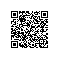# Androide SQLiteDatabase数据库操作(转)

SQLite可以解析大部分的标准SQL语句：

alter table 表名 add column 列名 字段类型

alter table 表名 rename to 新表名

SQLiteDatabase db=databaseHelper.getWritableDatabase();
db.execSQL(“update person set name=?,age=? where personid=?”,new Obect{person.getName(),person.getAge(),person.getId()});
execSQL()方法是用来执行除查找语句外的sql语句，查找语句用rawQuery()来执行它会放回一个Cursor。当然，他还提供了封装好的Java类方法供我们操作。具体方法不介绍了，可以直接查看文档中SQLiteDatabase类的用法。

ContentValues values=new ContentValues();
values.put(MyHelper.COUNTRY, "中国");
values.put(MyHelper.CODE,86);
//插入一行数据
db.insert(MyHelper.TD_NAME, MyHelper.ID, values);

String sql1="insert into "
+MyHelper.TD_NAME+"("
+MyHelper.COUNTRY+","
+MyHelper.CODE+")values"
+"(?,789)";
String[] data={"Japan"};
db.execSQL(sql1, data);

SQLiteDatabase db = this.getWritableDatabase();
String where = BOOK_ID + " = ?";
String[] whereValue = { Integer.toString(id) };

ContentValues cv = new ContentValues();
cv.put(BOOK_NAME, bookname);
cv.put(BOOK_AUTHOR, author);
db.update(TABLE_NAME, cv, where, whereValue);使用钉钉扫一扫加入圈子
+ 订阅